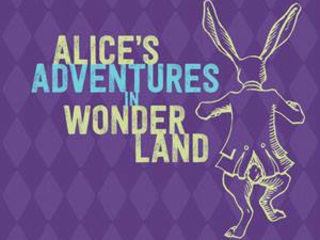# Harry Ransom Center presents Alice's Adventures in WonderlandThe Harry Ransom Center is celebrating 150 years of Alice's Adventures in Wonderland with an exhibition for all ages that only gets curious and curiouser.

Learn about Lewis Carroll and the real Alice who inspired his story, see one of the few surviving copies of the first edition of Alice's Adventures in Wonderland, discover the rich array of personal and literary references that Carroll incorporated throughout his work, follow the White Rabbit's path through the exhibition, have a tea party or watch a 1933 paper filmstrip that has been carefully treated by Ransom Center conservators. The Center's vast collection offers a new look at a story that has delighted generations and inspired artists from Salvador Dalí to Walt Disney.

The exhibit will run from February 10 until July 6.

### Event Details

#### When

7.6.15 | 9:00 am
7.4.15 | 9:00 am
7.3.15 | 9:00 am
+ see all dates and times
7.2.15 | 9:00 am
7.1.15 | 9:00 am
6.30.15 | 9:00 am
6.29.15 | 9:00 am
6.27.15 | 9:00 am
6.26.15 | 9:00 am
6.25.15 | 9:00 am
6.24.15 | 9:00 am
6.23.15 | 9:00 am
6.22.15 | 9:00 am
6.20.15 | 9:00 am
6.19.15 | 9:00 am
6.18.15 | 9:00 am
6.17.15 | 9:00 am
6.16.15 | 9:00 am
6.15.15 | 9:00 am
6.13.15 | 9:00 am
6.12.15 | 9:00 am
6.11.15 | 9:00 am
6.10.15 | 9:00 am
6.9.15 | 9:00 am
6.8.15 | 9:00 am
6.6.15 | 9:00 am
6.5.15 | 9:00 am
6.4.15 | 9:00 am
6.3.15 | 9:00 am
6.2.15 | 9:00 am
6.1.15 | 9:00 am
5.30.15 | 9:00 am
5.29.15 | 9:00 am
5.28.15 | 9:00 am
5.27.15 | 9:00 am
5.26.15 | 9:00 am
5.25.15 | 9:00 am
5.23.15 | 9:00 am
5.22.15 | 9:00 am
5.21.15 | 9:00 am
5.20.15 | 9:00 am
5.19.15 | 9:00 am
5.18.15 | 9:00 am
5.16.15 | 9:00 am
5.15.15 | 9:00 am
5.14.15 | 9:00 am
5.13.15 | 9:00 am
5.12.15 | 9:00 am
5.11.15 | 9:00 am
5.9.15 | 9:00 am
5.8.15 | 9:00 am
5.7.15 | 9:00 am
5.6.15 | 9:00 am
5.5.15 | 9:00 am
5.4.15 | 9:00 am
5.2.15 | 9:00 am
5.1.15 | 9:00 am
4.30.15 | 9:00 am
4.29.15 | 9:00 am
4.28.15 | 9:00 am
4.27.15 | 9:00 am
4.25.15 | 9:00 am
4.24.15 | 9:00 am
4.23.15 | 9:00 am
4.22.15 | 9:00 am
4.21.15 | 9:00 am
4.20.15 | 9:00 am
4.18.15 | 9:00 am
4.17.15 | 9:00 am
4.16.15 | 9:00 am
4.15.15 | 9:00 am
4.14.15 | 9:00 am
4.13.15 | 9:00 am
4.11.15 | 9:00 am
4.10.15 | 9:00 am
4.9.15 | 9:00 am
4.8.15 | 9:00 am
4.7.15 | 9:00 am
4.6.15 | 9:00 am
4.4.15 | 9:00 am
4.3.15 | 9:00 am
4.2.15 | 9:00 am
4.1.15 | 9:00 am
3.31.15 | 9:00 am
3.30.15 | 9:00 am
3.28.15 | 9:00 am
3.27.15 | 9:00 am
3.26.15 | 9:00 am
3.25.15 | 9:00 am
3.24.15 | 9:00 am
3.23.15 | 9:00 am
3.21.15 | 9:00 am
3.20.15 | 9:00 am
3.19.15 | 9:00 am
3.18.15 | 9:00 am
3.17.15 | 9:00 am
3.16.15 | 9:00 am
3.14.15 | 9:00 am
3.13.15 | 9:00 am
3.12.15 | 9:00 am
3.11.15 | 9:00 am
3.10.15 | 9:00 am
3.9.15 | 9:00 am
3.7.15 | 9:00 am
3.6.15 | 9:00 am
3.5.15 | 9:00 am
3.4.15 | 9:00 am
3.3.15 | 9:00 am
3.2.15 | 9:00 am
2.28.15 | 9:00 am
2.27.15 | 9:00 am
2.26.15 | 9:00 am
2.25.15 | 9:00 am
2.24.15 | 9:00 am
2.23.15 | 9:00 am
2.21.15 | 9:00 am
2.20.15 | 9:00 am
2.19.15 | 9:00 am
2.18.15 | 9:00 am
2.17.15 | 9:00 am
2.16.15 | 9:00 am
2.14.15 | 9:00 am
2.13.15 | 9:00 am
2.12.15 | 9:00 am
2.11.15 | 9:00 am
2.10.15 | 9:00 am

#### Where

Harry Ransom Center
300 W. 21st St. University of Texas at Austin
Austin, TX 78712